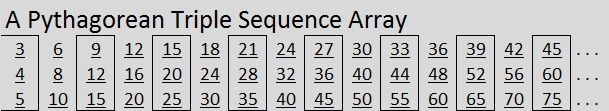# 368 and a Few Sequences

368 is a composite number because it is even. Scroll down for its factoring information.

Sequences can be illustrated in pictures, but they are most often simply a list of numbers such as 23, 46, 69, 92, 115, . . . . .

Those numbers are the multiples of 23 listed in order. If we continued writing the numbers in that sequence, we would see that 368 is the 16th number listed.

We also know that 368 is an element of many other sequences even though it isn’t one of the typed numbers:

• The sequence of all counting numbers: 1, 2, 3, 4, 5, . . . .
• The sequence of all even counting numbers: 2, 4, 6, 8, 10, . . . .
• The sequence of numbers that are multiples of four: 4, 8, 12, 16, 20, . . . . (This is the yellow sequence in the graphic below.)
• The sequence of numbers that are one less than the multiples of three: 2, 5, 8, 11, 14, . . . .
• The sequence of numbers that are one less than the multiples of nine: 8, 17, 26, 35, 44, . . . .386 is NOT an element of any of the following sequences that involve even squared numbers:

• 3, 15, 35, 63, 99, . . . . (The blue sequence in the graphic above)
• 4, 16, 36, 64, 100, . . . .
• 5, 17, 37, 65, 101, . . . . (The red sequence in the graphic above)

The yellow, blue, and red sequences above can be made into an array that contains only Primitive Pythagorean Triples!Except for the 3, 4, 5 triangle that is listed first, every multiple of 4 is the short leg in a primitive Pythagorean triple! The other leg and the hypotenuse are based on a square with sides that are 1/2 the length of the shorter leg. Since the shorter leg is a multiple of four, that square length will always be an even number. Also note: 368 is divisible by 4 so 368, 33855, 33857 is a primitive that would be included in this sequence array.

The sequence contains only primitives, but it does NOT contain EVERY primitive whose short leg is a multiple of four. For example Pythagorean triple, 20, 21, 29, is not in that sequence array.

Let’s look at all the factoring information for 368:

• 368 is a composite number.
• Prime factorization: 368 = 2 x 2 x 2 x 2 x 23, which can be written 368 = (2^4) x 23
• The exponents in the prime factorization are 4 and 1. Adding one to each and multiplying we get (4 + 1)(1 + 1) = 5 x 2 = 10. Therefore 368 has exactly 10 factors.
• Factors of 368: 1, 2, 4, 8, 16, 23, 46, 92, 184, 368
• Factor pairs: 368 = 1 x 368, 2 x 184, 4 x 92, 8 x 46, or 16 x 23
• Taking the factor pair with the largest square number factor, we get √368 = (√16)(√23) = 4√23 ≈ 19.183We can make a sequence array from the Pythagorean triple primitive 3, 4, 5 and its non-primitive multiples (which includes 276, 368, 460):We can find other Pythagorean triples that contain the number 368. Notice that 368 has several factors that are multiples of 4. They are 4, 8, 16, 92, and 184. Each one of them has its own primitive triple which can be multiplied by its factor pair partner to produce non-primitive triples that include 368 as well.

• 3, 4, 5 multiplied by 92 is 276, 368, 460
• 8, 15, 17 multiplied by 46 is 368, 690, 782
• 16, 63, 65 multiplied by 23 is 368, 1449, 1495
• 92, 2115, 2117 multiplied by 4 is 368, 8460, 8468
• 184, 8463, 8465 multiplied by 2 is 368, 16926, 16930

In future posts I’ll write about how to find other Pythagorean triples like

• 23, 264, 265, a primitive Pythagorean triple with an odd short leg. This primitive becomes 368, 4224, 4240 when multiplied by 16 (23’s factor pair partner).
• 368, 465, 593, another primitive Pythagorean triple not included in the colored sequence array above and
• 184, 513, 545, which when multiplied by 2 becomes 368, 1026, 1090.

Pythagorean triples aren’t just a bunch of “SQUARE” numbers. They are simply counting numbers that satisfy the equation a² + b² = c², and they are really COOL!